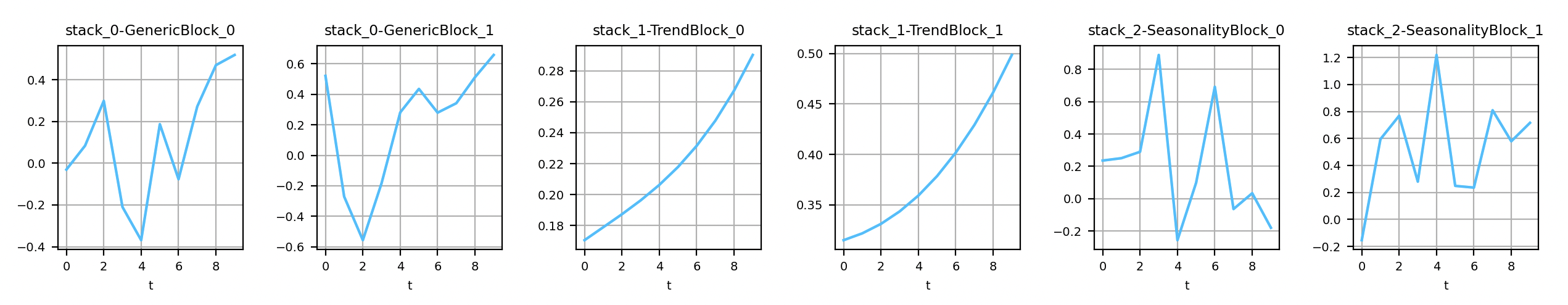# N Beats

Keras/Pytorch implementation of N-BEATS: Neural basis expansion analysis for interpretable time series forecasting.
Alternatives To N Beats
Darts5,940716 hours ago25June 22, 2022237apache-2.0Python
A python library for user-friendly forecasting and anomaly detection on time series.
Tsai3,49516 days ago41April 19, 202232apache-2.0Jupyter Notebook
Time series Timeseries Deep Learning Machine Learning Pytorch fastai | State-of-the-art Deep Learning library for Time Series and Sequences in Pytorch / fastai
Pytorch Forecasting2,85845 days ago33May 23, 2022391mitPython
Time series forecasting with PyTorch
Deep Learning Time Series1,811
9 months ago8apache-2.0Jupyter Notebook
List of papers, code and experiments using deep learning for time series forecasting
Awesome_time_series_in_python1,811
4 months ago4
This curated list contains python packages for time series analysis
Flow Forecast1,460
3 days ago94gpl-3.0Python
Deep learning PyTorch library for time series forecasting, classification, and anomaly detection (originally for flood forecasting).
Pmdarima1,3646457 days ago21February 22, 202242mitPython
A statistical library designed to fill the void in Python's time series analysis capabilities, including the equivalent of R's auto.arima function.
Pytorch Ts958
2 months ago9April 24, 202258mitPython
PyTorch based Probabilistic Time Series forecasting framework based on GluonTS backend
Autots74713 days ago40June 20, 20227mitPython
Automated Time Series Forecasting
N Beats686
3 months agomitPython
Keras/Pytorch implementation of N-BEATS: Neural basis expansion analysis for interpretable time series forecasting.
Alternatives To N Beats
Select To Compare

Alternative Project Comparisons

## NBEATSNeural basis expansion analysis for interpretable time series forecasting

Tensorflow/Pytorch implementation | Paper | ResultsOutputs of the generic and interpretable layers of NBEATS

### Installation

It is possible to install the two backends at the same time.

#### From PyPI

Install the Tensorflow/Keras backend: `pip install nbeats-keras`Install the Pytorch backend: `pip install nbeats-pytorch`#### From the sources

Installation is based on a MakeFile.

Command to install N-Beats with Keras: `make install-keras`

Command to install N-Beats with Pytorch: `make install-pytorch`

#### Run on the GPU

This trick is no longer necessary on the recent versions of Tensorflow. To force the utilization of the GPU (with the Keras backend), run: `pip uninstall -y tensorflow && pip install tensorflow-gpu`.

### Example

Here is an example to get familiar with both backends. Note that only the Keras backend supports `input_dim>1` at the moment.

``````import warnings

import numpy as np

from nbeats_keras.model import NBeatsNet as NBeatsKeras
from nbeats_pytorch.model import NBeatsNet as NBeatsPytorch

warnings.filterwarnings(action='ignore', message='Setting attributes')

def main():
# https://keras.io/layers/recurrent/
# At the moment only Keras supports input_dim > 1. In the original paper, input_dim=1.
num_samples, time_steps, input_dim, output_dim = 50_000, 10, 1, 1

# This example is for both Keras and Pytorch. In practice, choose the one you prefer.
for BackendType in [NBeatsKeras, NBeatsPytorch]:
# NOTE: If you choose the Keras backend with input_dim>1, you have
# to set the value here too (in the constructor).
backend = BackendType(
backcast_length=time_steps, forecast_length=output_dim,
stack_types=(NBeatsKeras.GENERIC_BLOCK, NBeatsKeras.GENERIC_BLOCK),
nb_blocks_per_stack=2, thetas_dim=(4, 4), share_weights_in_stack=True,
hidden_layer_units=64
)

# Definition of the objective function and the optimizer.

# Definition of the data. The problem to solve is to find f such as | f(x) - y | -> 0.
# where f = np.mean.
x = np.random.uniform(size=(num_samples, time_steps, input_dim))
y = np.mean(x, axis=1, keepdims=True)

# Split data into training and testing datasets.
c = num_samples // 10
x_train, y_train, x_test, y_test = x[c:], y[c:], x[:c], y[:c]
test_size = len(x_test)

# Train the model.
print('Training...')
backend.fit(x_train, y_train, validation_data=(x_test, y_test), epochs=20, batch_size=128)

# Save the model for later.
backend.save('n_beats_model.h5')

# Predict on the testing set (forecast).
predictions_forecast = backend.predict(x_test)
np.testing.assert_equal(predictions_forecast.shape, (test_size, backend.forecast_length, output_dim))

# Predict on the testing set (backcast).
predictions_backcast = backend.predict(x_test, return_backcast=True)
np.testing.assert_equal(predictions_backcast.shape, (test_size, backend.backcast_length, output_dim))

np.testing.assert_almost_equal(predictions_forecast, model_2.predict(x_test))

if __name__ == '__main__':
main()
``````

Browse the examples for more. It includes Jupyter notebooks.

Jupyter notebook: NBeats.ipynb: `make run-jupyter`.

### Citation

``````@misc{NBeatsPRemy,
author = {Philippe Remy},
title = {N-BEATS: Neural basis expansion analysis for interpretable time series forecasting},
year = {2020},
publisher = {GitHub},
journal = {GitHub repository},
howpublished = {\url{https://github.com/philipperemy/n-beats}},
}
``````

### Contributors

Thank you!Popular Forecasting Projects
Popular Series Projects
Popular Machine Learning Categories
Related Searches

Get A Weekly Email With Trending Projects For These Categories
No Spam. Unsubscribe easily at any time.
Python
Deep Learning
Pytorch
Neural Network
Neural
Keras
Series
Time Series
Forecasting